# Math in Focus Grade 2 Chapter 11 Practice 1 Answer Key Coins and Bills

Practice the problems of Math in Focus Grade 2 Workbook Answer Key Chapter 11 Practice 1 Coins and Bills to score better marks in the exam.

## Math in Focus Grade 2 Chapter 11 Practice 1 Answer Key Coins and Bills

Circle the bills that make the given amount.

Example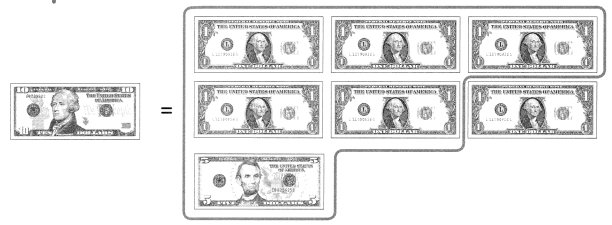Question 1.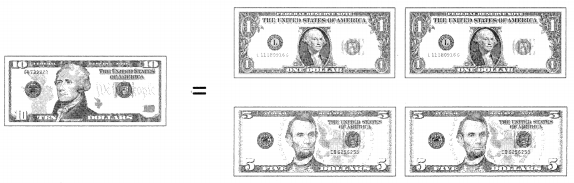10 dollar = 1 d0llar + 1 dollar + 5 dollar + 5 dollar.
10 dollars = 12 dollars.
Then circle the 10 d0llars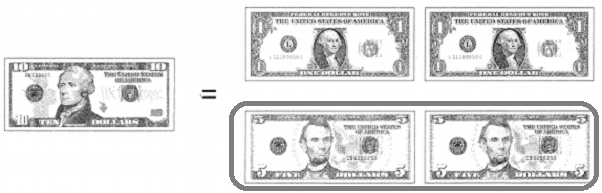Question 2.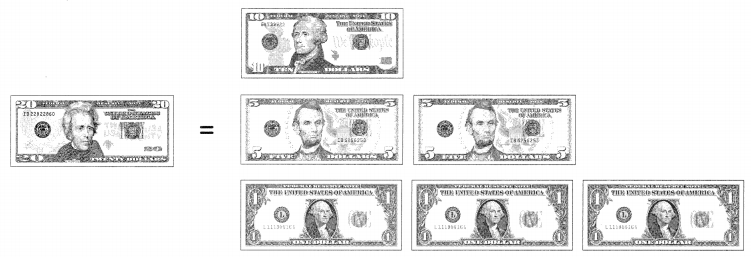20 dollars = 10 dollars + 5 dollars + 5 dollars + 1 dollar + 1 dollar + 1 dollar.
20 dollars = 23 dollars.
Then circle the 20 dollars.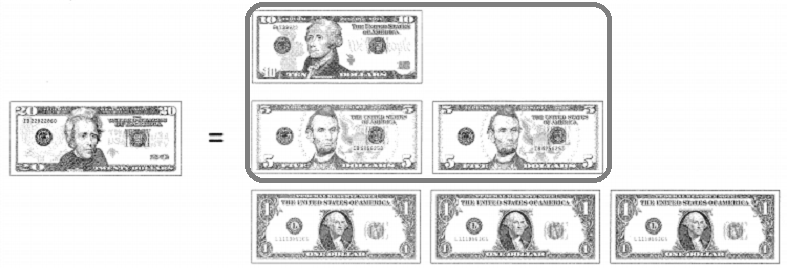Circle the coins that make one dollar.

Question 3.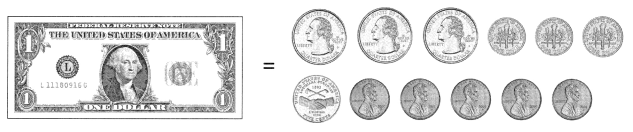1 dollar = 100 cents
The circle part is equal to 100 cents.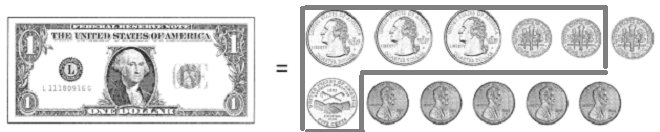Question 4.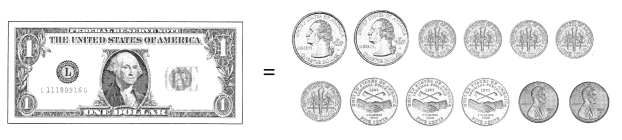1 dollar = 100 cents
The circle part is equal to 100 cents.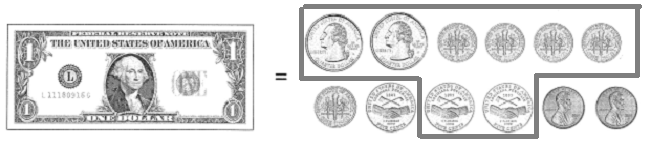Find the value of the coins. Then write less than, equal to, or more than.

Example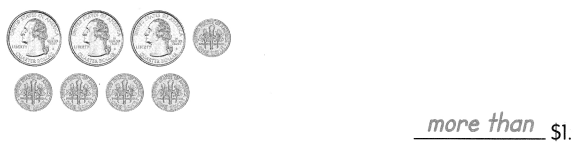Question 5.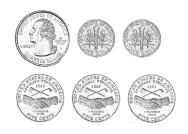___________ $1 Answer: 1 Quarter + 10 cents + 10cents + 5 cents + 5 cents. 1 quarter = 25 cents 25 cents + 10 cents + 10cents + 5 cents + 5 cents = 55cents 55 cents less than$1.

Question 6.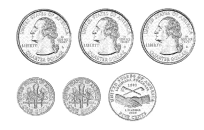___________ $1 Answer: Quarter + quarter + quarter + 10 cents + 10 cents + 5 cents. 1 quarter = 25 cents. 25 cents + 25 cents + 25 cents + 10 cents + 10 cents + 5 cents = 100 cents. 100 cents equal to$1

Question 7.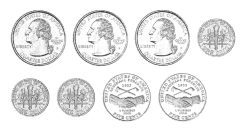___________ $1 Answer: Quarter + quarter + quarter + 10 cents + 10 cents + 10 cents + 5 cents + 5 cents. 1 quarter = 25 cents. 25 cents + 25 cents + 25 cents + 10 cents + 10 cents + 10 cents + 5 cents + 5 cents = 115 cents. 100 cents =$1
115 cents more than to $1 Write the amount of money. Example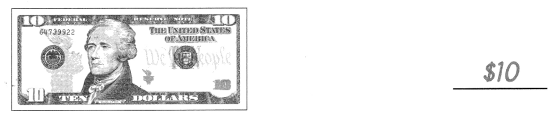Question 8.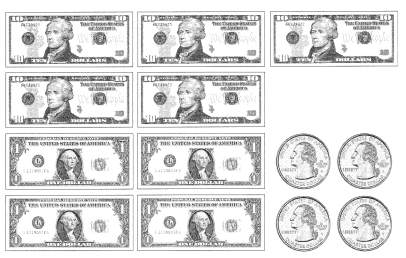$_____________
Answer: $10 +$10 + $10 +$10 + $10 +$1 + $1 +$1 + $1 + 1 quarter + 1 quarter + 1 quarter + 1 quarter. 1 quarter = 25 cents. 4 quarters =$1
$10 +$10 + $10 +$10 + $10 +$1 + $1 +$1 + $1 +$1 = $55 Question 9.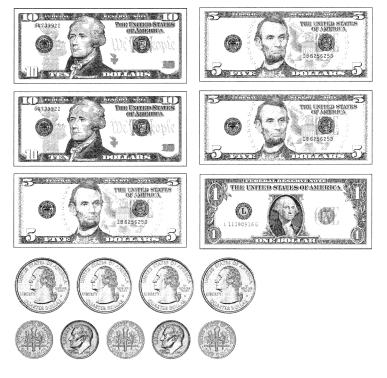$_____________
$10 +$10 + $5 +$5 + $5 +$1 + 1 quarter + 1 quarter + 1 quarter + 1 quarter + 10 cents + 1 dime + 10 cents + 1 dime + 10 cents.
1 quarter = 25 cents.
1 dime = 10 cents.
$10 +$10 + $5 +$5 + $5 +$1 + 25 cents  + 25 cents + 25 cents + 25 cents + 10 cents + 10 cents + 10 cents + 10 cents + 10 cents = $37.50 Write the amount of money. Example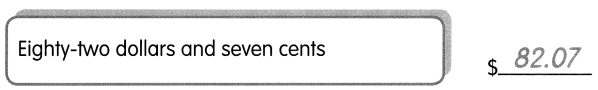Question 10.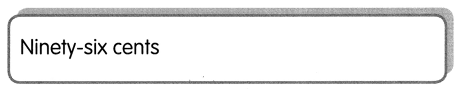$_____________
1 cent = 0.01 dollar.
Ninety six cents = $0.96 Question 11.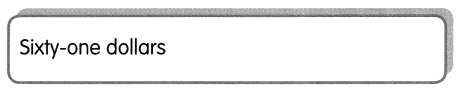$_____________
Sixty one dollars = $61 Write the amount of money. Question 12.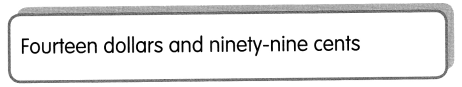$_____________
1 cent = 0.01 dollar.
99 cents = 0.99 dollars.
Fourteen dollars and ninety nine cents = $14.99 Question 13.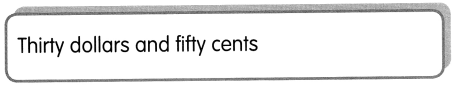$_____________
1 cent = 0.01 dollar
50 cents = 0.50 d0llar.

Thirty dollars and fifty cents = $13.50 Question 14.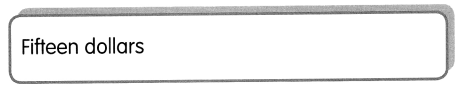$_____________
Fifteen dollars = $15 Question 15.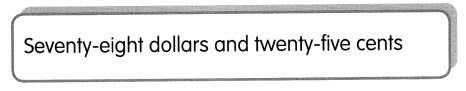$_____________
1 cent = 0.01 dollar.
25 cents = 0.25 dollar.
Seventy eight dollars and twenty five cents = $78.25 Fill in the blanks. Example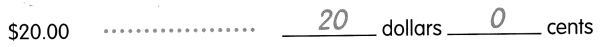Question 16.$.03 ………………….
__________ dollars __________ cents
$.03$0.03 dollars 0 cents

Question 17.
$40.20…………………. __________ dollars __________ cents Answer:$40.20 = $40 dollars 20 cents Question 18.$27.15 ………………….
__________ dollars __________ cents
$27.15 =$27 dollars 15 cents

Match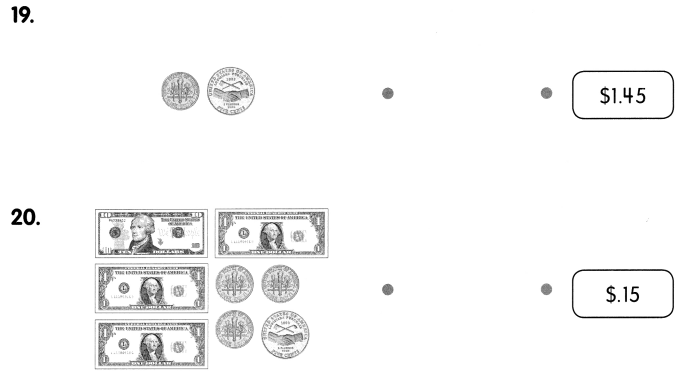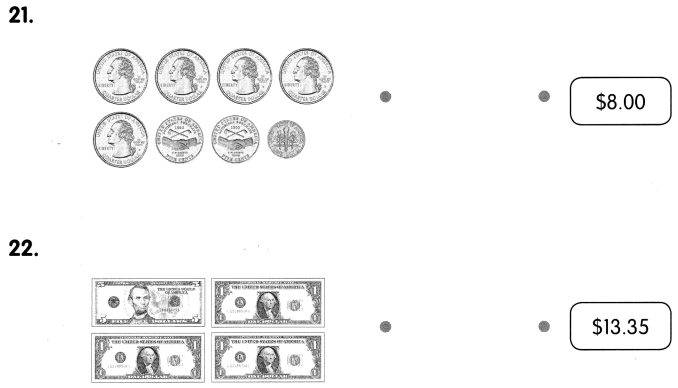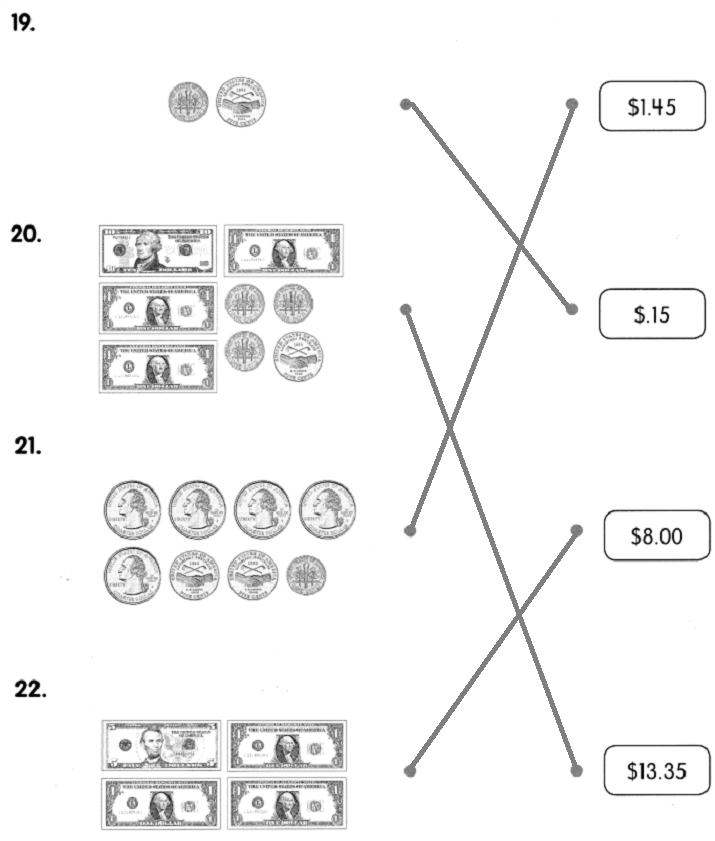Count the money. Then circle the correct amount.

Example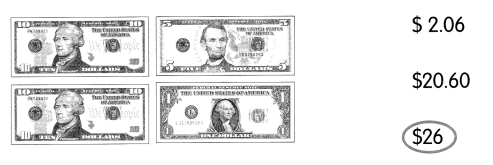Question 23.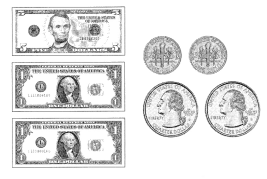$7.00$7.07
$7.70 Answer: 5 dollars + 1 dollar + 1 dollar + 10 cents + 10 cents + 1 quarter + 1 quarter. 1 quarter = 25 cents. The total money is$7.70
The correct money is $7.70 Question 24.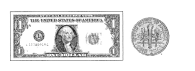$1.10
$11.00$11
1 dollar + 10 cents = $1.10 Question 25.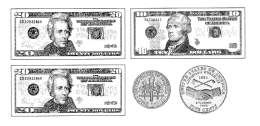$50.15
$.65$5.15
20 dollar + 20 dollar + 10 dollar + 10 cents + 5 cents = $50.15 Complete. Example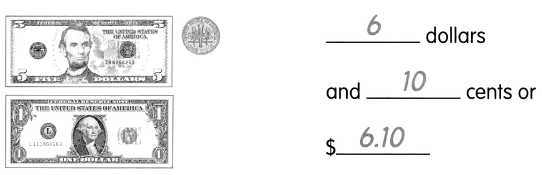Question 26.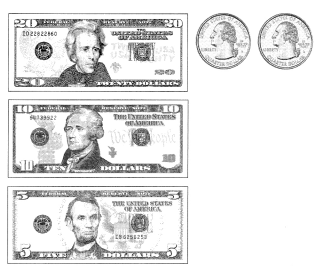__________ dollars and __________ cents or$__________
20 dollars + 10 dollars + 5 dollars + 1 quarter + 1 quarter = 35 dollars and 50 cents or $35.50 Question 27.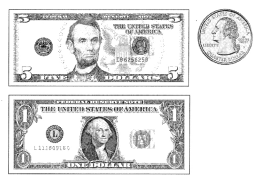__________ dollars and __________ cents or$__________
5 dollars + 1 dollar + 1 quarter = 6 dollars and 25 cents or $6.25 Question 28.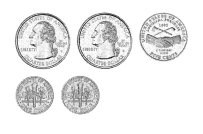__________ dollars and __________ cents or$__________
1 quarter + 1 quarter  + 5 cents + 10 cents + 10 cents = 0 dollars and 75 cents or $0.75 Question 29.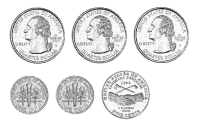__________ dollars and __________ cents or$__________
1 quarter + 1 quarter + 1 quarter + 10 cents + 10 cents + 5 cents = 1 dollars and 0 cents or $1.00 Question 30.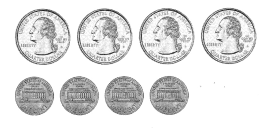__________ dollars and __________ cents or$__________
1 quarter + 1 quarter + 1 quarter + 1 quarter + 1 cent + 1 cent + 1 cent + 1 cent = 1 dollars and 4 cents or $1.04 Write the amount of money in two ways. Example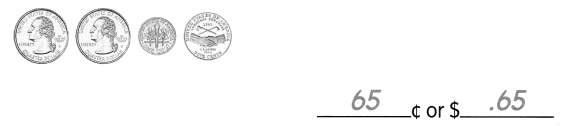Question 31.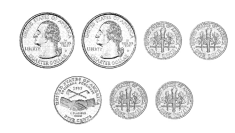____________¢ or$____________
1 quarter + 1 quarter + 10 cents + 10 cents + 5 cents + 10 cents + 10 cents = 95 ¢ or $0.95 Question 32.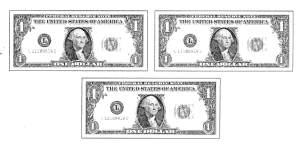____________¢ or$____________
1 dollar + 1 dollar + 1 dollar = 300 ¢ or $3.00 Question 33.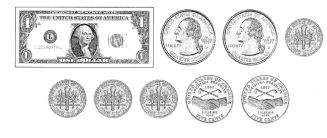____________¢ or$____________
1 dollar + 1 quarter + 1 quarter + 10 cents + 10 cents + 10 cents + 10 cents + 5 cents + 5 cents = 200 ¢ or $2.00 Write the amount of money in two ways. Example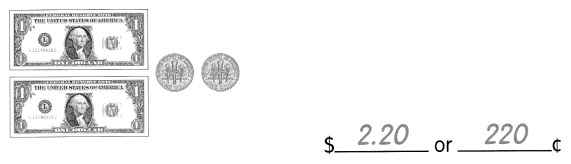Question 34.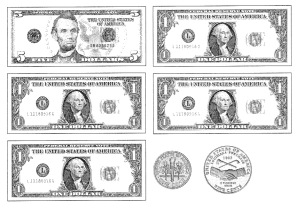$___________ or ____________¢
5 dollars + 1 dollar + 1 dollar + 1 dollar + 1 dollar + 10 cents + 5 cents = $9.15 or 915¢ Question 35.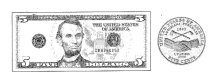$___________ or ____________¢
5 dollars + 5 cents = $5.05 or 505¢ Question 36.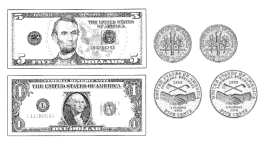$___________ or ____________¢
5 dollars + 1 dollar + 10 cents + 10 cents + 5 cents + 5 cents = $6.30 or 630¢ Write the cents in dollars and cents. Example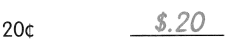Question 37. 120¢ ___________ Answer: 100 cents = 1 dollar 120 cents =1.20$

Question 38.
543¢ ____________
100 cents = 1 dollar
543 cents = $5.43 Question 39. 106¢ _____________ Answer: 100 cents = 1 dollar 106 cents =$1.06

Question 40.
350¢ ____________
1dollar = 100 cents
3 dollar = 300 cents
300cents + 50 cents = $3.50 Question 41. 83¢ ___________ Answer: 1 cent = 0.01 dollar 83 cents =$0.83

Question 42.
17¢ ____________
1 cent = 0.01 dollar
17 cents = $0.17 Question 43. 2¢ ____________ Answer: 1 cent = 0.01 dollar 2 cents =$0.02

Write the dollars and cents in cents.

Example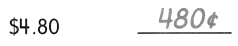Question 44.
$3.51 ____________ Answer: 1 dollar = 100 cents 3 dollar = 300 cents 300 cents = 51 cents = 351¢ Question 45.$6.95 ____________
1 dollar = 100 cents
6 dollars = 600 cents
600 cents + 95 cents = 695¢

Question 46.
$1.05 ____________ Answer: 1 dollar = 100 cents$1.05 =105¢

Question 47.
$.44 ____________ Answer: 1 cent = 0.01 dollar$.44 = 44¢

Question 48.
$.69 ____________ Answer: 1 cent = 0.01 dollar 69 cents = 0.69 cents = 69¢ Question 49.$8 ____________